# NCERT Exemplar Class 9 Science Solutions for Chapter 10 - Gravitation

## NCERT Exemplar Solutions Class 9 Science Chapter 10 – Free PDF Download

NCERT Exemplar Solutions for Class 9 Science Gravitation is an essential study material for the students who wish to score well in Class 9 examination. This Exemplar page provides a variety of question with varied difficulty. Solving these NCERT Exemplars will help to understand the concepts clearly and this will, in turn, help you to take a note of key concepts.

The NCERT Exemplar Class 9 Science questions are designed in such a way that students can only answer these questions if they have properly read the chapter and understood all the concepts of gravitation. They have 15 multiple choice questions, 7 short answer questions and 4 long answer questions. Visit the below link to get free NCERT Exemplar for Class 9 Science Chapter 9.

### Download PDF of NCERT Exemplar for Class 9 Science Chapter 10 – Gravitation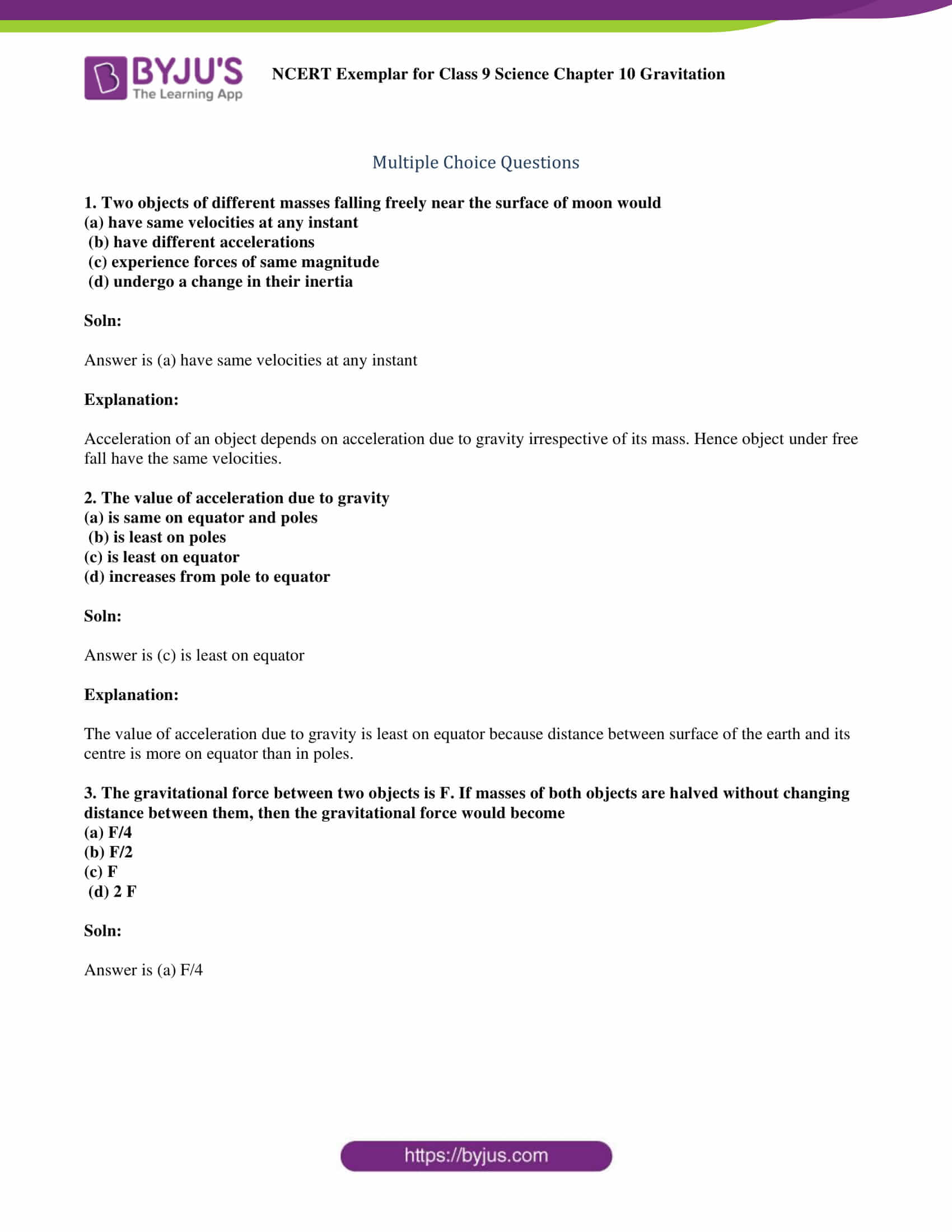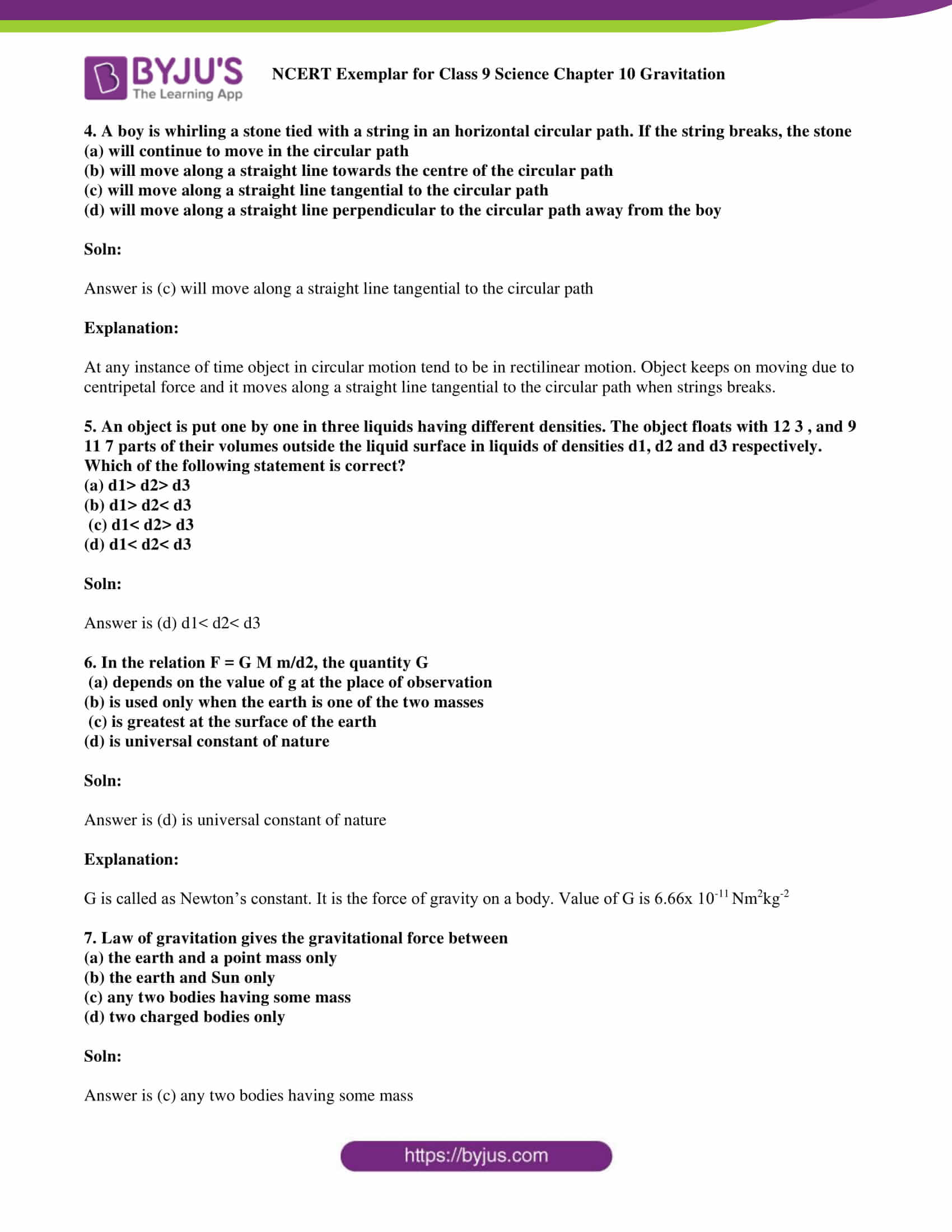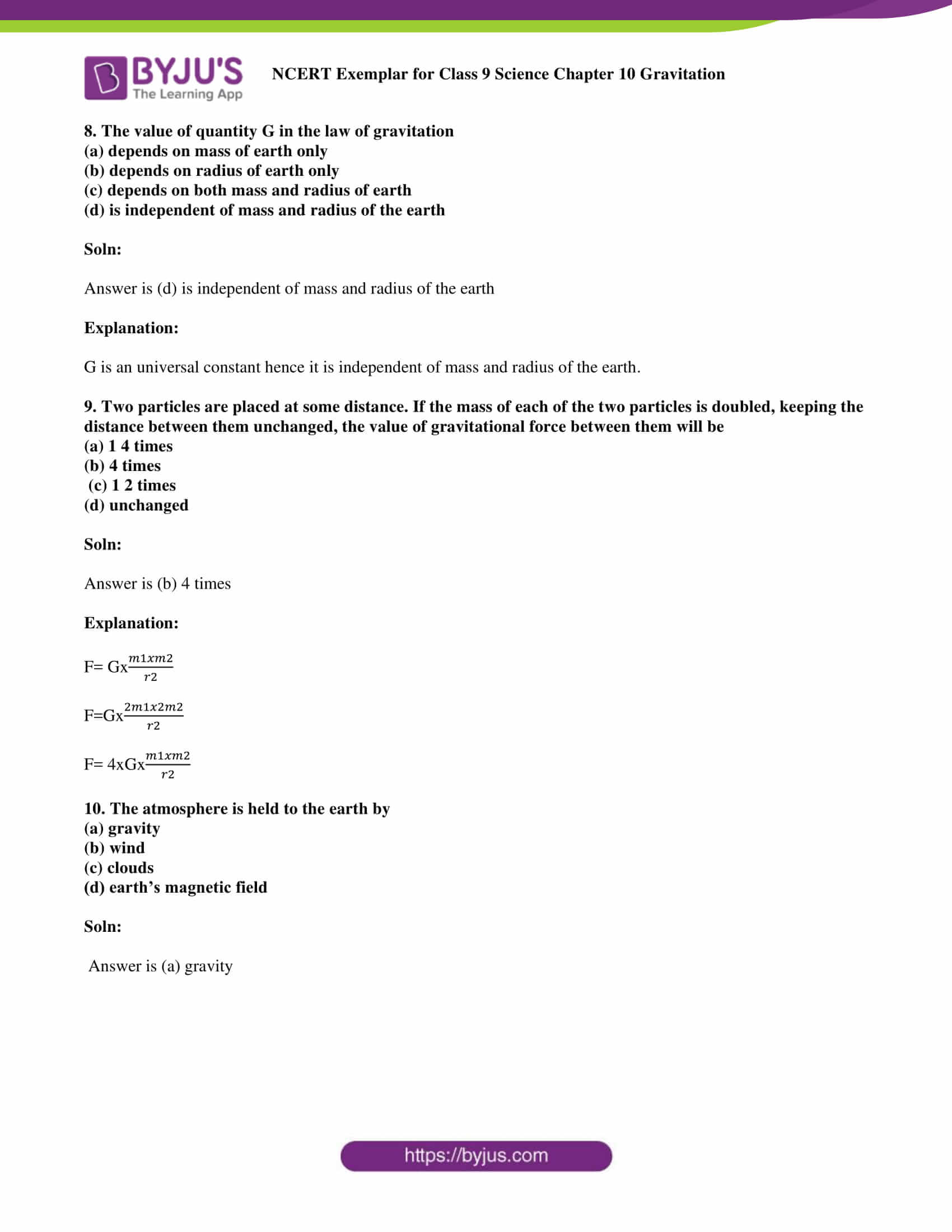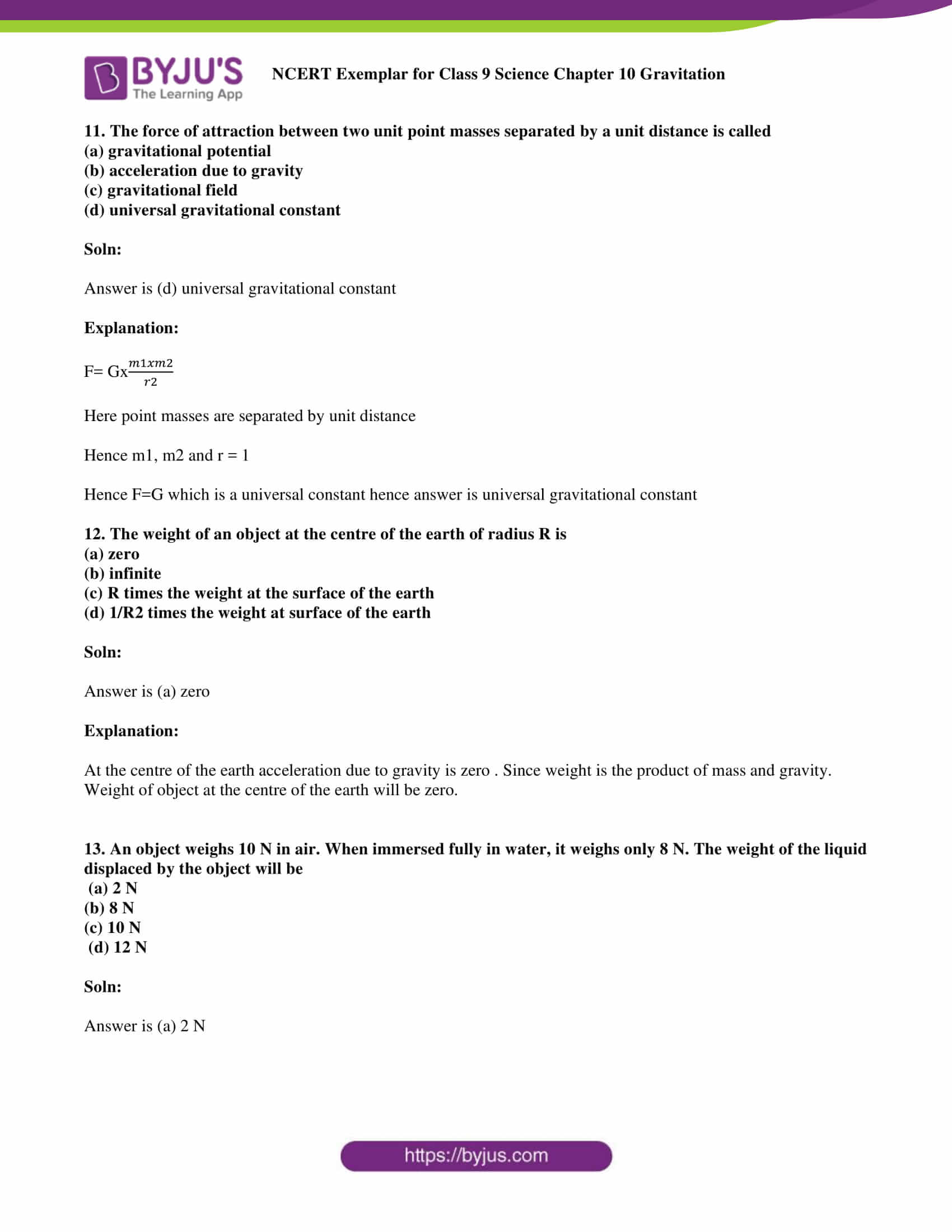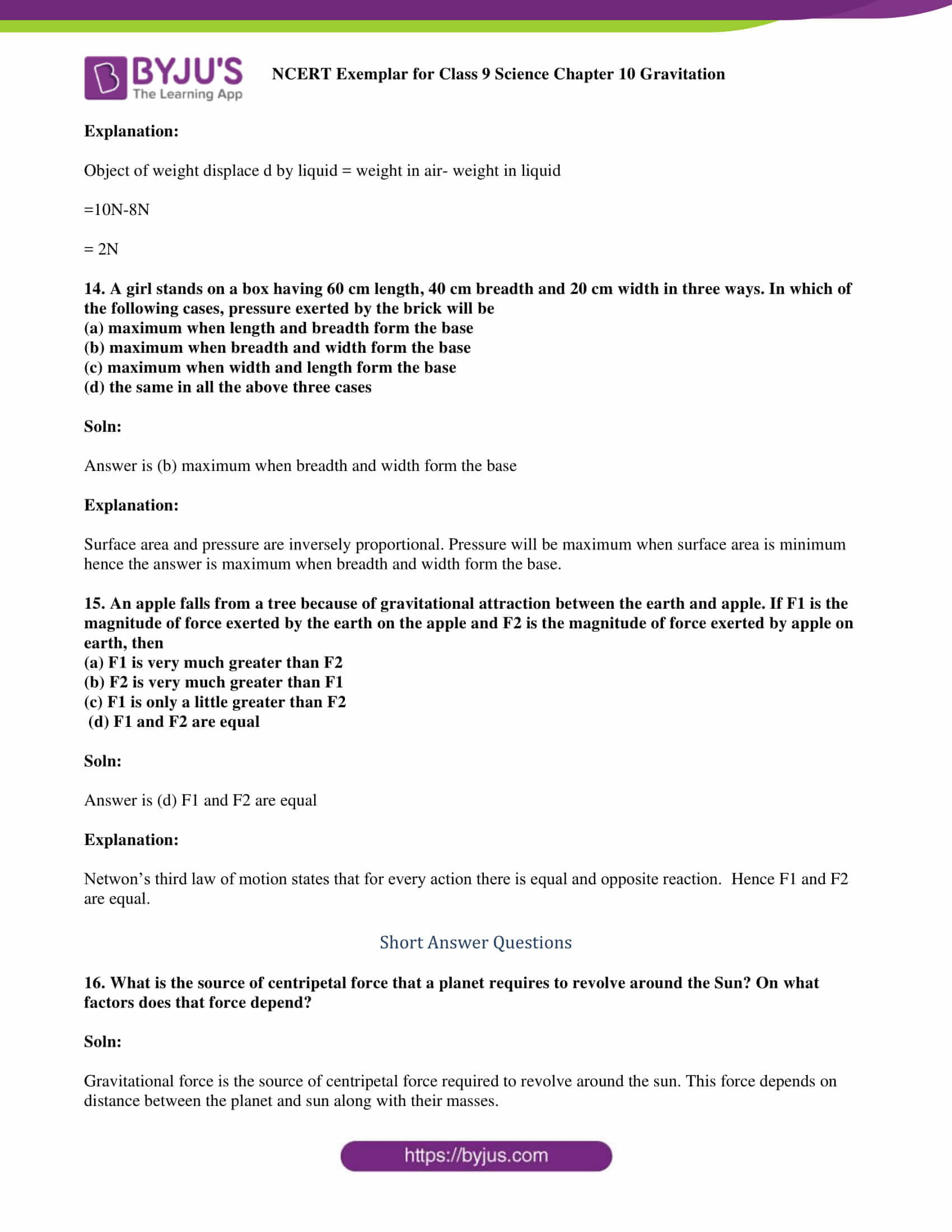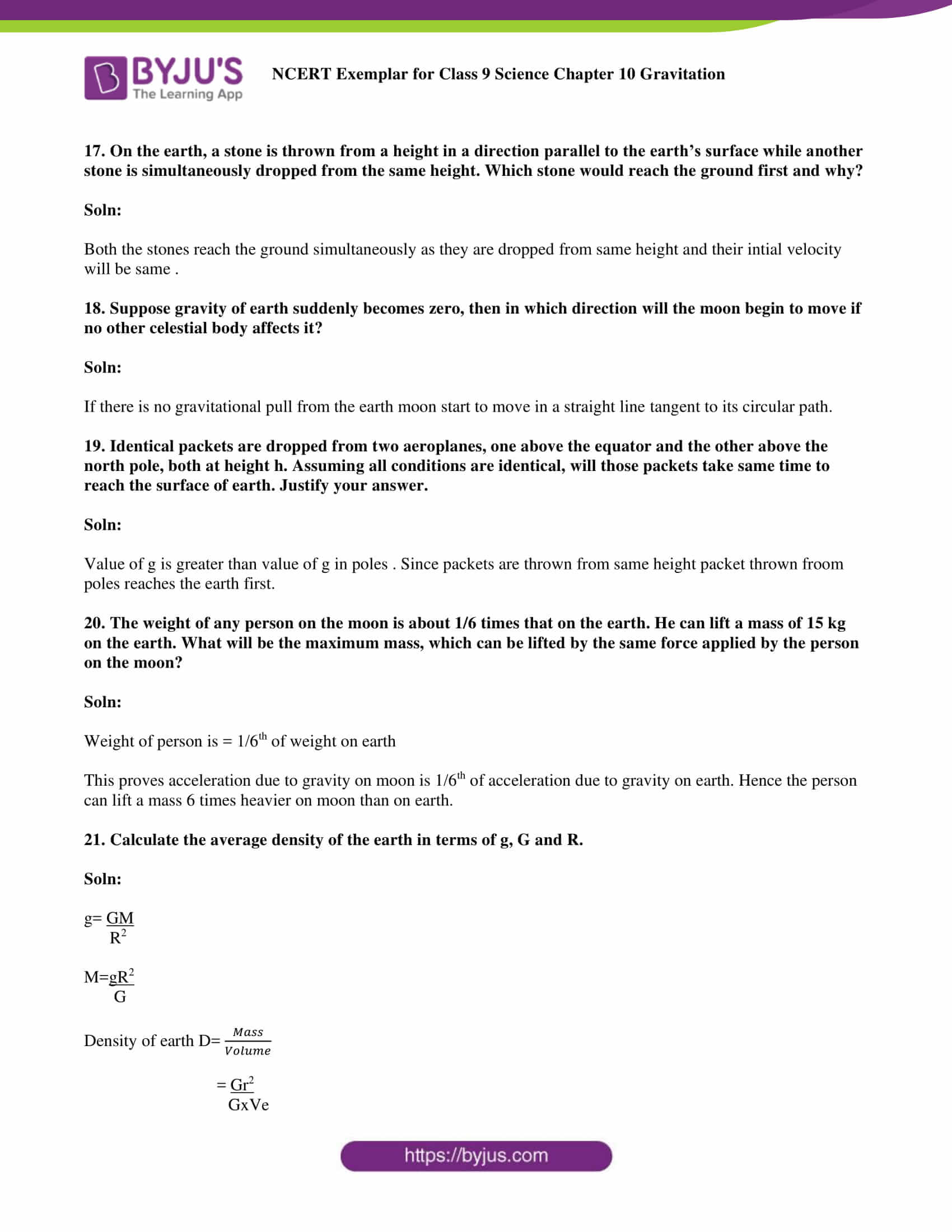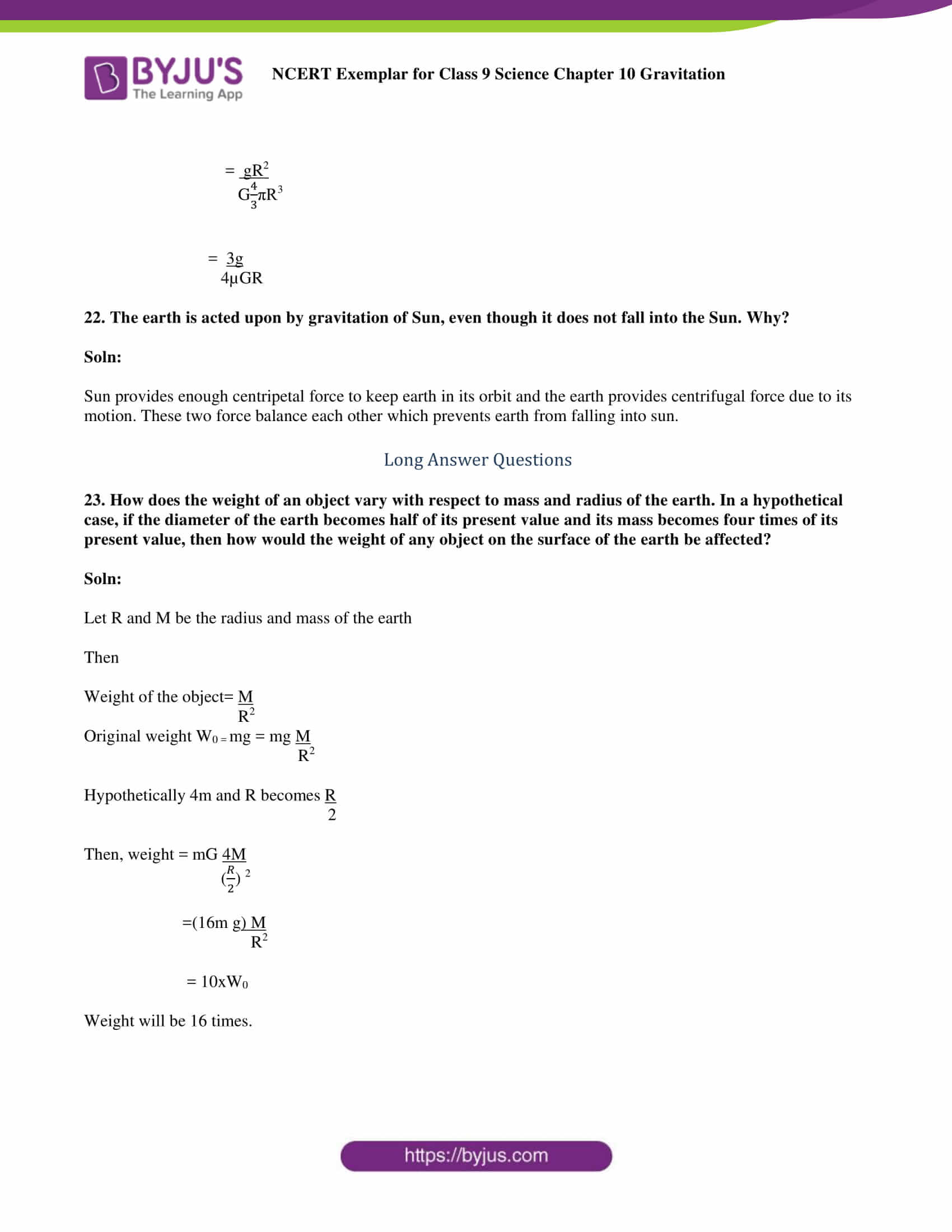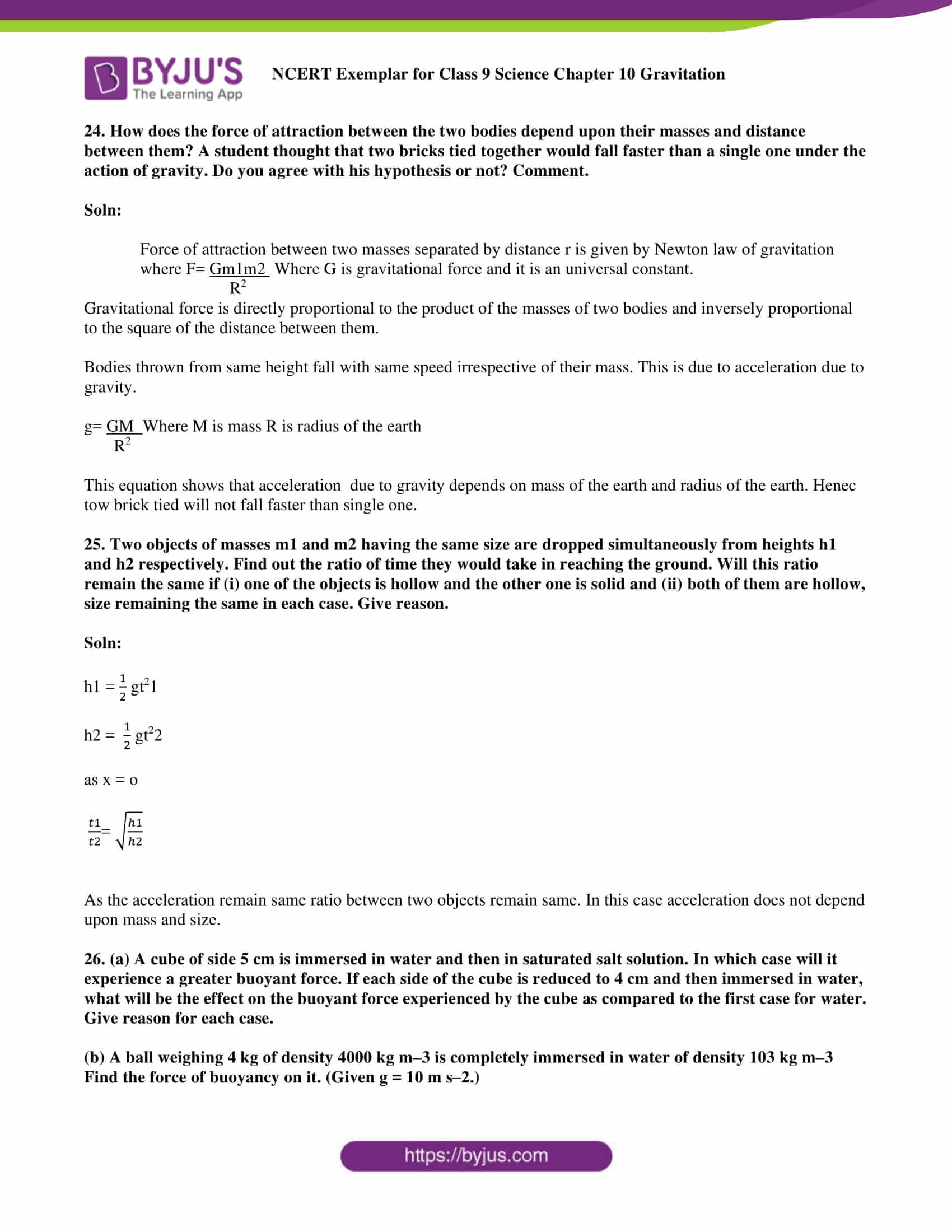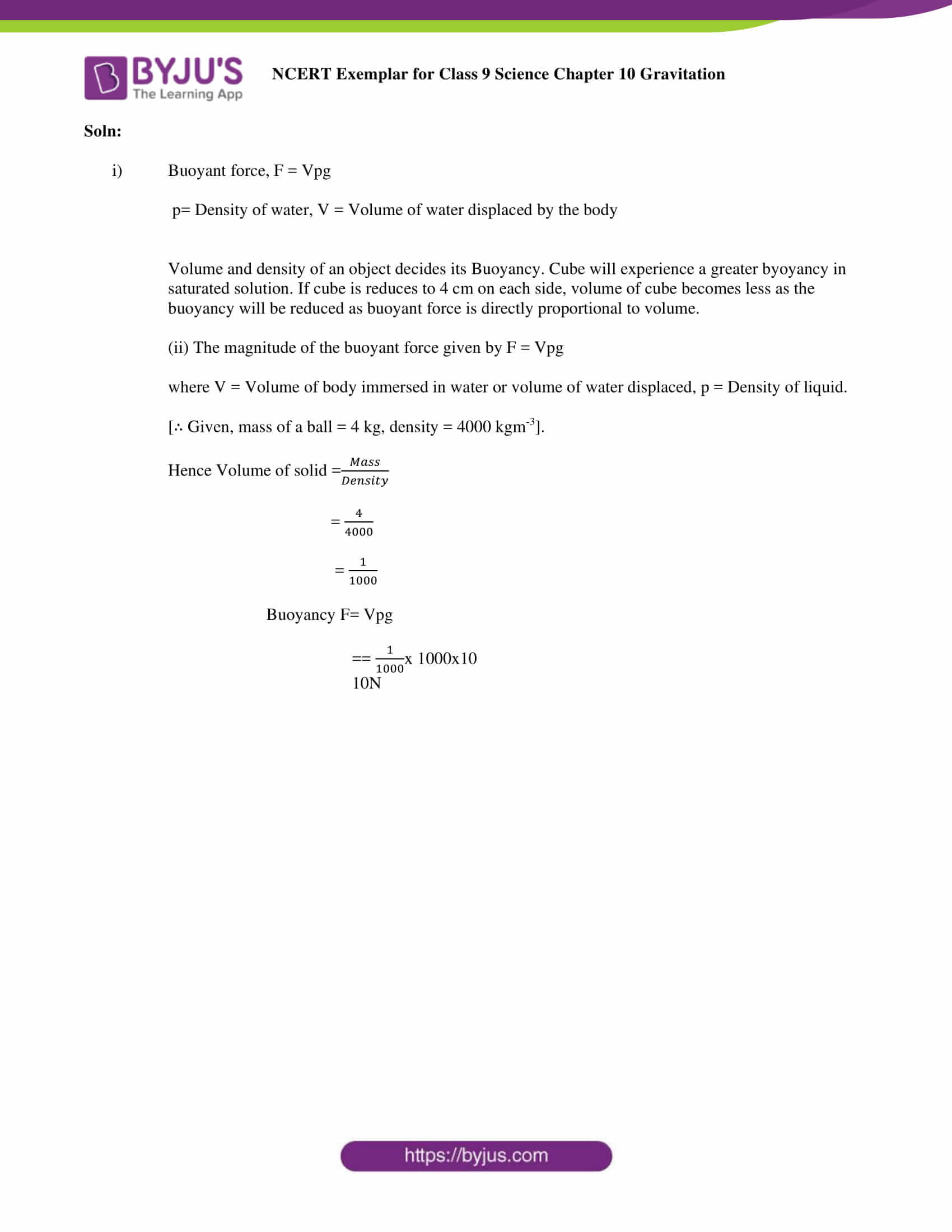### Multiple Choice Questions

1. Two objects of different masses falling freely near the surface of moon would

(a) have same velocities at any instant

(b) have different accelerations

(c) experience forces of same magnitude

(d) undergo a change in their inertia

Soln:

Answer is (a) have same velocities at any instant

Explanation:

Acceleration of an object depends on acceleration due to gravity irrespective of its mass. Hence object under free fall have the same velocities.

2. The value of acceleration due to gravity

(a) is same on equator and poles

(b) is least on poles

(c) is least on equator

(d) increases from pole to equator

Soln:

Answer is (c) is least on equator

Explanation:

The value of acceleration due to gravity is least on equator because distance between surface of the earth and its centre is more on equator than in poles.

3. The gravitational force between two objects is F. If masses of both objects are halved without changing distance between them, then the gravitational force would become

(a) F/4

(b) F/2

(c) F

(d) 2 F

Soln:

4. A boy is whirling a stone tied with a string in an horizontal circular path. If the string breaks, the stone

(a) will continue to move in the circular path

(b) will move along a straight line towards the centre of the circular path

(c) will move along a straight line tangential to the circular path

(d) will move along a straight line perpendicular to the circular path away from the boy

Soln:

Answer is (c) will move along a straight line tangential to the circular path

Explanation:

At any instance of time object in circular motion tend to be in rectilinear motion. Object keeps on moving due to centripetal force and it moves along a straight line tangential to the circular path when strings breaks.

5. An object is put one by one in three liquids having different densities. The object floats with 12 3 , and 9 11 7 parts of their volumes outside the liquid surface in liquids of densities d1, d2 and d3 respectively. Which of the following statement is correct?

(a) d1> d2> d3

(b) d1> d2< d3

(c) d1< d2> d3

(d) d1< d2< d3

Soln:

Answer is (d) d1< d2< d3

6. In the relation F = G M m/d2, the quantity G

(a) depends on the value of g at the place of observation

(b) is used only when the earth is one of the two masses

(c) is greatest at the surface of the earth

(d) is universal constant of nature

Soln:

Answer is (d) is universal constant of nature

Explanation:

G is called as Newton’s constant. It is the force of gravity on a body. Value of G is 6.66x 10-11 Nm2kg-2

7. Law of gravitation gives the gravitational force between

(a) the earth and a point mass only

(b) the earth and Sun only

(c) any two bodies having some mass

(d) two charged bodies only

Soln:

Answer is (c) any two bodies having some mass

8. The value of quantity G in the law of gravitation

(a) depends on mass of earth only

(b) depends on radius of earth only

(c) depends on both mass and radius of earth

(d) is independent of mass and radius of the earth

Soln:

Answer is (d) is independent of mass and radius of the earth

Explanation:

G is an universal constant hence it is independent of mass and radius of the earth.

9. Two particles are placed at some distance. If the mass of each of the two particles is doubled, keeping the distance between them unchanged, the value of gravitational force between them will be

(a) 14 times

(b) 4 times

(c) 12 times

(d) unchanged

Soln:

10. The atmosphere is held to the earth by

(a) gravity

(b) wind

(c) clouds

(d) earth’s magnetic field

Soln:

11. The force of attraction between two unit point masses separated by a unit distance is called

(a) gravitational potential

(b) acceleration due to gravity

(c) gravitational field

(d) universal gravitational constant

Soln:

Answer is (d) universal gravitational constant

Explanation:

F= G x

$$\begin{array}{l}\frac{m1×m2}{r^{2}}\end{array}$$

Here point masses are separated by unit distance

Hence m1, m2 and r = 1

Hence F = G which is a universal constant hence answer is universal gravitational constant

12. The weight of an object at the centre of the earth of radius R is

(a) zero

(b) infinite

(c) R times the weight at the surface of the earth

(d) 1/R2 times the weight at surface of the earth

Soln:

Explanation:

At the centre of the earth acceleration due to gravity is zero. Since weight is the product of mass and gravity. Weight of the object at the centre of the earth will be zero.

13. An object weighs 10 N in air. When immersed fully in water, it weighs only 8 N. The weight of the liquid displaced by the object will be

(a) 2 N

(b) 8 N

(c) 10 N

(d) 12 N

Soln:

Explanation:

Object of weight displace d by liquid = weight in air- weight in liquid

=10N-8N

= 2N

14. A girl stands on a box having 60 cm length, 40 cm breadth and 20 cm width in three ways. In which of the following cases, pressure exerted by the brick will be

(a) maximum when length and breadth form the base

(b) maximum when breadth and width form the base

(c) maximum when width and length form the base

(d) the same in all the above three cases

Soln:

Explanation:

Surface area and pressure are inversely proportional. Pressure will be maximum when surface area is minimum hence the answer is maximum when breadth and width form the base.

15. An apple falls from a tree because of gravitational attraction between the earth and apple. If F1 is the magnitude of force exerted by the earth on the apple and F2 is the magnitude of force exerted by apple on earth, then

(a) F1 is very much greater than F2

(b) F2 is very much greater than F1

(c) F1 is only a little greater than F2

(d) F1 and F2 are equal

Soln:

Answer is (d) F1 and F2 are equal

Explanation:

Netwon’s third law of motion states that for every action there is equal and opposite reaction. Hence F1 and F2 are equal.

16. What is the source of centripetal force that a planet requires to revolve around the Sun? On what factors does that force depend?

Soln:

Gravitational force is the source of centripetal force required to revolve around the sun. This force depends on the distance between the planet and sun along with their masses. If this force becomes zero as a result of the absence of centripetal force, the planet would shift to moving tangentially outwards to the circular route.

17. On the earth, a stone is thrown from a height in a direction parallel to the earth’s surface while another stone is simultaneously dropped from the same height. Which stone would reach the ground first and why?

Soln:

Both the stones reach the ground simultaneously as they are dropped from the same height and their initial velocity will be the same.

18. Suppose gravity of earth suddenly becomes zero, then in which direction will the moon begin to move if no other celestial body affects it?

Soln:

If there is no gravitational pull from the earth, the moon starts to move in a straight line tangent to its circular path.

19. Identical packets are dropped from two aeroplanes, one above the equator and the other above the north pole, both at height h. Assuming all conditions are identical, will those packets take same time to reach the surface of earth. Justify your answer.

Soln:

The value of ‘g’ – acceleration due to gravity is constant, but depending upon the surface of the earth it varies from place to place as earth is not completely spherical. As it is flattened at the poles, the value of ‘g’ is maximum at the poles and the bulging at the equator causes the ‘g’ value to be minimum at the equator. The ‘g’ value increases as we move towards the poles. Hence, the packets falls gradually at the equator in comparison to the poles. Thereby, the packets stay in air for longer when dropped at the equator.

20. The weight of any person on the moon is about 1/6 times that on the earth. He can lift a mass of 15 kg on the earth. What will be the maximum mass, which can be lifted by the same force applied by the person on the moon?

Soln:

Weight of person on moon = 1/6th of weight on earth

Therefore, ‘g’ on moon = 1/6th ‘g’ on earth

The force that is applied by the man to lift mass ‘m’ is

F = mg = 15g    (on earth)

If he can lift a certain mass ‘m’ by applying the same force on moon, then

F = 15*6=90kg

This proves acceleration due to gravity on the moon is 1/6th of acceleration due to gravity on earth. Hence the person can lift a mass 6 times heavier on moon than on earth.

21. Calculate the average density of the earth in terms of g, G and R.

Soln:

g= GM /R2

M=gR2/G

Density of earth D= mass/volume

=Gr2/GxVe

= gR2/G4πR/3

= 3g/4µGR

22. The earth is acted upon by gravitation of Sun, even though it does not fall into the Sun. Why?

Soln:

Sun provides enough centripetal force to keep the earth in its orbit and the earth provides centrifugal force due to its motion. These two forces balance each other which prevents the earth from falling into the sun.

23. How does the weight of an object vary with respect to mass and radius of the earth. In a hypothetical case, if the diameter of the earth becomes half of its present value and its mass becomes four times of its present value, then how would the weight of any object on the surface of the earth be affected?

Soln:

Let R and M be the radius and mass of the earth

Then

Weight of the object = M/R2

Original weight W0 = mg = mg M/R2

Hypothetically 4m and R becomes R/2

Then, weight = mG 4M /(R/2) 2

=(16m g) M/R2

= 10xW0

Weight will be 16 times.

24. How does the force of attraction between the two bodies depend upon their masses and distance between them? A student thought that two bricks tied together would fall faster than a single one under the action of gravity. Do you agree with his hypothesis or not? Comment.

Soln:

The hypothesis is incorrect. Force of attraction between two masses separated by distance r is given by Newton law of gravitation where F= Gm1m2/R2 Where G is gravitational force and it is an universal constant.

Gravitational force is directly proportional to the product of the masses of two bodies and inversely proportional to the square of the distance between them.

Bodies thrown from same height fall with same speed irrespective of their mass. This is due to acceleration due to gravity.

g = GM/R2Where M is mass R is radius of the earth

This equation shows that acceleration due to gravity depends on the mass of the earth and radius of the earth. Hence tow brick tied will not fall faster than the single one.

25. Two objects of masses m1 and m2 having the same size are dropped simultaneously from heights h1 and h2 respectively. Find out the ratio of time they would take in reaching the ground. Will this ratio remain the same if (i) one of the objects is hollow and the other one is solid and (ii) both of them are hollow, size remaining the same in each case. Give reason.

Soln:

h1 =

$$\begin{array}{l}\frac{1}{2}\end{array}$$
gt21

h2 =

$$\begin{array}{l}\frac{1}{2}\end{array}$$
gt22

as x = o

$$\begin{array}{l}\frac{t1}{t2}\end{array}$$
2 = √h1/h2

As the acceleration remains the same, the ratio between two objects remains the same. In this case, acceleration does not depend upon mass and size.

26. (a) A cube of side 5 cm is immersed in water and then in saturated salt solution. In which case will it experience a greater buoyant force. If each side of the cube is reduced to 4 cm and then immersed in water, what will be the effect on the buoyant force experienced by the cube as compared to the first case for water. Give reason for each case.

(b) A ball weighing 4 kg of density 4000 kg m–3 is completely immersed in water of density 103 kg m–3 Find the force of buoyancy on it. (Given g = 10 m s–2)

Soln:

(i) Buoyant force, F = Vpg

p = Density of water, V = Volume of water displaced by the body

Volume and density of an object decides its Buoyancy. Cube will experience a greater buoyancy in a saturated solution. If the cube is reduced to 4 cm on each side, the volume of cube becomes less as the buoyancy will be reduced as buoyant force is directly proportional to volume.

(ii) The magnitude of the buoyant force given by F = Vpg

where V = Volume of body immersed in water or volume of water displaced, p = Density of liquid.

[∴ Given, mass of a ball = 4 kg, density = 4000 kgm-3].

Hence Volume of solid =

$$\begin{array}{l}\frac{mass}{density}\end{array}$$

=

$$\begin{array}{l}\frac{4}{4000}\end{array}$$

=

$$\begin{array}{l}\frac{1}{1000}\end{array}$$

Buoyancy F = Vpg

=

$$\begin{array}{l}\frac{1}{1000}\end{array}$$
x 1000×10

= 10N

### Topics Involved in NCERT Exemplar for Chapter 10 Gravitation

1. Gravitation
2. Importance of the universal law of gravitation
1. To calculate the value of g
2. The motion of objects under the influence of the gravitational force of the
3. Mass-Weight
1. The weight of an object on the moon
4. Thrust and Pressure
1. Pressure in fluids
2. Buoyancy
3. Why objects float or sink when placed on the surface of the water?
5. Archimedes’ Principle
6. Relative Density.

With BYJU’S students can excel in their exam. BYJU’S modern learning approach will guide you in understanding the concepts clearly and also helps you in solving the questions easily. You can get all the study materials along with NCERT Exemplar provided by us by registering with BYJU’S or by downloading BYJU’S Learning App.

## Frequently Asked Questions on NCERT Exemplar Solutions for Class 9 Science Chapter 10

### Is the BYJU’S NCERT Exemplar Solutions for Class 9 Science Chapter 10 available in PDF format?

Yes, BYJU’S provides the NCERT Exemplar Solutions for Class 9 Science Chapter 10 in PDF format which can be downloaded by the students. These reference materials from BYJU’S are hassle free and help students to score well in the Class 9 exams. The PDF’s created by the experts are of high quality and the free download links can be used by the students to access them.

### What are the uses of referring to BYJU’S NCERT Exemplar Solutions for Class 9 Science Chapter 10?

The uses of referring to BYJU’S NCERT Exemplar Solutions for Class 9 Science Chapter 10 are –
1. Each answer has a comprehensive explanation to help students understand the concepts.
2. The solutions are strictly based on the syllabus designed by the CBSE board.
3. The chapter wise solutions are accurate and genuine to improve the problem solving skills among students.

### What are the topics covered in the NCERT Exemplar Solutions for Class 9 Science Chapter 10?

The topics covered in the NCERT Exemplar Solutions for Class 9 Science Chapter 10 are –
1. Gravitation
2. Importance of the universal law of gravitation
a. To calculate the value of g
b. The motion of objects under the influence of the gravitational force of the
3. Mass-Weight
a. The weight of an object on the moon
4. Thrust and Pressure
a. Pressure in fluids
b. Buoyancy
c. Why do objects float or sink when placed on the surface of the water?
5. Archimedes’ Principle
6. Relative Density.